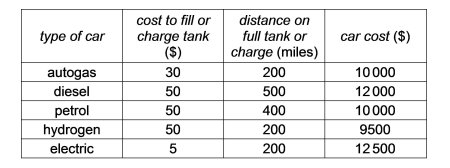# IMAT 2020 Q9 [New Car]

Jeff is looking to buy a new car. He will only be using it for commuting to his workplace for 1 year and will have a total annual mileage of 20000. He wants to buy the car which would make his total spend over the year, including the initial cost of the car, the lowest.Using the table above, which type of car should he buy?

A. diesel
B. hydrogen
C. petrol
D. autogas
E. electric

1 Like

Simple steps to solve word problems:

• Underline key information
• Determine what they are trying to ask, and what you will need to solve it
• Eliminate any non-essential information
• Draw a picture, graph, or equation
• In moments of high stress like exam taking, always work with the paper they give you to avoid careless mistakes.
• Solve.

This is a longer question that is best saved for the end of the exam after you have grabbed the easy points. To solve it, we need to factor in the car cost, and the amount spent on fuel for each type of car.

We can make a general equation and then plug everything in.

Total cost = car cost + (cost to fuel)(\\$20 000 miles/distance till fuel needed)

A diesel

Total cost = \\$12 000 + (\\$50)(20 000 miles/500 miles)
Total cost = \\$12 000 + (\\$50)(40)
Total cost = \\$12 000 + (\\$2000)
Total cost = \\$14 000

B hydrogen

Total cost = \\$9 500 + (\\$50)(20 000 miles/200 miles)
Total cost = \\$9 500 + (\\$50)(100)
Total cost = \\$9 500 + (\\$5000)
Total cost = \\$14 500

C petrol

Total cost = \\$10 000 + (\\$50)(20 000 miles/400 miles)
Total cost = \\$10 000 + (\\$50)(50)
Total cost = \\$10 000 + (\\$2500)
Total cost = \\$12 500

D autogas

Total cost = \\$10 000 + (\\$30)(20 000 miles/200 miles)
Total cost = \\$10 000 + (\\$30)(100)
Total cost = \\$10 000 + (\\$3000)
Total cost = \\$13 000

E electric

Total cost = \\$12 500 + (\\$5)(20 000 miles/200 miles)
Total cost = \\$12 500 + (\\$5)(100)
Total cost = \\$12 500 + (\\$500)
Total cost = \\$13 000

As we can see, the petrol car will be the cheapest for Jeff’s needs.

\fcolorbox{red}{grey!30}{Therefore the answer is C, Petrol.}